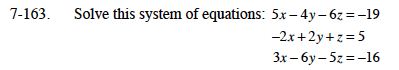Home > CCA2 > Chapter 7 > Lesson 7.2.4 > Problem7-163

7-163.
1. Solve this system of equations: Homework Help ✎

2. 5x − 4y − 6z = −19
−2x + 2y + z = 5
3x − 6y − 5z = −16Use the Elimination Method to eliminate y from the first and second equations.

Use the Elimination Method to eliminate y from the second and third equations.

Use the two new equations you have formed to solve for x and z. Remember to solve for y as well.